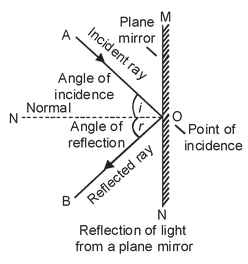# Some Important Terms Used In The Reflection Of Light

## SOME IMPORTANT TERMS USED IN THE REFLECTION OF LIGHT

Let us consider the reflection of light by a plane mirror as shown in the diagram.

### INCIDENT RAY:

The ray of light that strikes the surface is called the incident ray. In the below figure ‘AO’ is the incident ray.

### POINT OF INCIDENCE:

The point of incidence is that point at which light is incident on the reflecting surface. i.e., the point at which the incident ray falls on the reflecting surface.

In below figure ‘O’ is the point of incidence.### NORMAL:

The perpendicular drawn to the reflecting surface at the point of incidence is called normal. In the above figure, ‘ON’ is the normal.

### REFLECTED RAY:

The ray of light which is turned back after reflection into the same medium in which the incident light is travelling is called the reflected ray.

In above figure OB is the reflected ray.

### ANGLE OF INCIDENCE:

The angle of incidence is the angle between a ray incident on a surface and the line perpendicular to the surface at the point of incidence, called thenormal.

Or

The angle between the incident ray and the normal to the surface is called angle of incidence. In the above figure, ∠AON is the angle of incidence.

### ANGLE OF REFLECTION:

The angle formed by a reflected ray and a perpendicular to the surface at the point of reflection.

Or

The angle between the reflected ray and normal to the surface is called the angle of reflection. In the above figure, ∠BON is the angle of reflection.